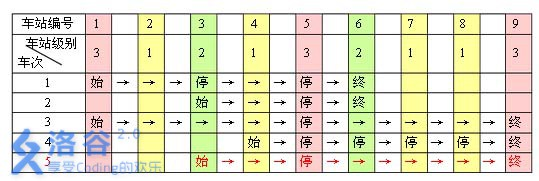# P1983 车站分级

• 4.7K通过
• 15.8K提交
• 题目提供者 CCF_NOI
• 评测方式 云端评测
• 标签 图的建立,建图 图论 拓扑排序 排序 贪心 NOIp普及组 2013 O2优化 高性能
• 难度 提高+/省选-
• 时空限制 1000ms / 128MB
• 提示：收藏到任务计划后，可在首页查看。

## 题目描述

一条单向的铁路线上，依次有编号为 $1, 2, …, n$的 $n$个火车站。每个火车站都有一个级别，最低为 $1$ 级。现有若干趟车次在这条线路上行驶，每一趟都满足如下要求：如果这趟车次停靠了火车站 $x$，则始发站、终点站之间所有级别大于等于火车站$x$ 的都必须停靠。（注意：起始站和终点站自然也算作事先已知需要停靠的站点）

例如，下表是$5$趟车次的运行情况。其中，前$4$ 趟车次均满足要求，而第 $5$ 趟车次由于停靠了 $3$ 号火车站（$2$ 级）却未停靠途经的 $6$ 号火车站（亦为 $2$ 级）而不满足要求。现有 $m$ 趟车次的运行情况（全部满足要求），试推算这$n$ 个火车站至少分为几个不同的级别。

## 输入输出格式

输入格式：

第一行包含 $2$ 个正整数 $n, m$，用一个空格隔开。

第 $i + 1$ 行$(1 ≤ i ≤ m)$中，首先是一个正整数 $s_i(2 ≤ s_i ≤ n)$，表示第$i$ 趟车次有 $s_i$ 个停靠站；接下来有$s_i$个正整数，表示所有停靠站的编号，从小到大排列。每两个数之间用一个空格隔开。输入保证所有的车次都满足要求。

输出格式：

一个正整数，即 $n$ 个火车站最少划分的级别数。

## 输入输出样例

输入样例#1： 复制
9 2
4 1 3 5 6
3 3 5 6 
输出样例#1： 复制
2
输入样例#2： 复制
9 3
4 1 3 5 6
3 3 5 6
3 1 5 9 
输出样例#2： 复制
3

## 说明

对于$20\%$的数据，$1 ≤ n, m ≤ 10$；

对于 $50\%$的数据，$1 ≤ n, m ≤ 100$；

对于 $100\%$的数据，$1 ≤ n, m ≤ 1000$。

提示
标程仅供做题后或实在无思路时参考。
请自觉、自律地使用该功能并请对自己的学习负责。
如果发现恶意抄袭标程，将按照I类违反进行处理。